Open in App
Not now

# How to Place Legend Outside of the Plot in Matplotlib?

• Difficulty Level : Basic
• Last Updated : 26 Nov, 2022

In this article, we will see how to put the legend outside the plot.

Let’s discuss some concepts :

• Matplotlib: Matplotlib is an amazing visualization library in Python for 2D plots of arrays. Matplotlib is a multi-platform data visualization library built on NumPy arrays and designed to work with the broader SciPy stack. It was introduced by John Hunter in the year 2002.
• Legend: A legend is an area describing the elements of the graph. In the Matplotlib library, there’s a function called legend() which is used to Place a legend on the axes. The attribute Loc in legend() is used to specify the location of the legend. The default value of loc is loc=  “best” (upper left).

## Put the legend outside the plot

As, we can see that the above figure legends overlapped on the graph i.e; incomplete information. To solve this problem we need to place the legend outside the plot.

### The syntax to set the legend outside is as given below:

matplotlib.pyplot.legend(bbox_to_anchor=(x,y))

### Example 1:

Matplotlib set legend upper-left outside the plot.

## Python3

 `import` `matplotlib.pyplot as plt` `import` `numpy as np`   `x ``=` `np.linspace(``0``, ``10``, ``100``)`   `plt.plot(x, np.sin(x), label``=``"sin(x)"``)` `plt.plot(x, np.cos(x), label``=``"cos(x)"``)`     `plt.legend(bbox_to_anchor``=``(``1.05``, ``1.0``), loc``=``'upper left'``)` `plt.tight_layout()` `plt.show()`

Output :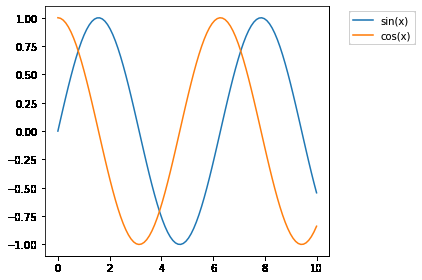### Example 2:

Matplotlib set legend center-left outside the plot.

## Python3

 `import` `matplotlib.pyplot as plt` `import` `numpy as np`   `x ``=` `np.linspace(``0``, ``10``, ``100``)`   `plt.plot(x, np.sin(x), label``=``"sin(x)"``)` `plt.plot(x, np.cos(x), label``=``"cos(x)"``)`     `plt.legend(bbox_to_anchor ``=` `(``1.25``, ``0.6``), loc``=``'center right'``)` `plt.tight_layout()` `plt.show()`

Output: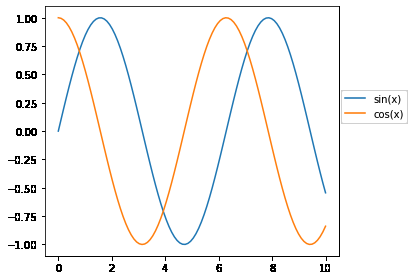### Example 3:

Matplotlib set legend lower-right outside the plot.

## Python3

 `import` `matplotlib.pyplot as plt` `import` `numpy as np`   `x ``=` `np.linspace(``0``, ``10``, ``100``)`   `plt.plot(x, np.sin(x), label``=``"sin(x)"``)` `plt.plot(x, np.cos(x), label``=``"cos(x)"``)`     `plt.legend(bbox_to_anchor ``=``(``1.44``,``-``0.10``), loc``=``'lower right'``)` `plt.tight_layout()` `plt.show()`

Output: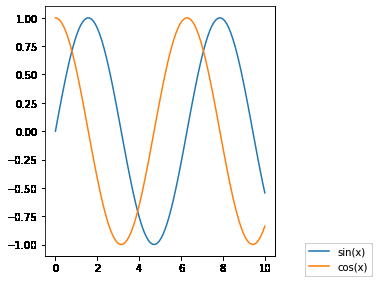### Example 4:

Matplotlib set legend upper-center outside the plot.

## Python3

 `import` `matplotlib.pyplot as plt` `import` `numpy as np`   `x ``=` `np.linspace(``0``, ``10``, ``100``)`   `plt.plot(x, np.sin(x), label``=``"sin(x)"``)` `plt.plot(x, np.cos(x), label``=``"cos(x)"``)`     `plt.legend(bbox_to_anchor``=``(``0.5``, ``1.2``), loc``=``'upper center'``)` `plt.tight_layout()` `plt.show()`

Output :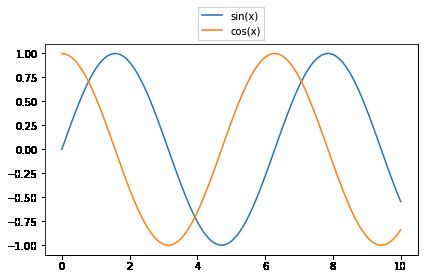### Example 5:

Matplotlib set legend lower-center outside the plot

## Python3

 `import` `matplotlib.pyplot as plt` `import` `numpy as np`   `x ``=` `np.linspace(``0``, ``10``, ``100``)`   `plt.plot(x, np.sin(x), label``=``"sin(x)"``)` `plt.plot(x, np.cos(x), label``=``"cos(x)"``)`     `plt.legend(bbox_to_anchor ``=``(``0.5``,``-``0.27``), loc``=``'lower center'``)` `plt.tight_layout()` `plt.show()`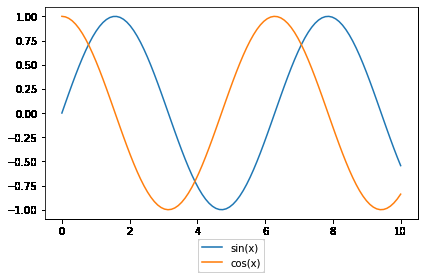### Example 6:

Place with subplots Legend Outside of the Plot

## Python3

 `# importing packages` `import` `numpy as np` `import` `matplotlib.pyplot as plt`   `# create data` `x ``=` `np.linspace(``-``5``, ``5``, ``1000``)` `colors``=``[[``'c'``,``'g'``], [``'y'``,``'r'``]]`   `# make subplot and plots the graphs` `fig, ax ``=` `plt.subplots(``2``, ``2``)` `for` `i ``in` `range``(``2``):` `    ``ax[``0``][i].plot(x, np.sin(x``+``i),` `                  ``color ``=` `colors[``0``][i],` `                  ``label ``=` `"y=sin(x+{})"``.``format``(i))` `    `  `    ``ax[``1``][i].plot(x, np.sin(x``+``i), ` `                  ``color ``=` `colors[``1``][i],` `                  ``label ``=` `"y=sin(x+{})"``.``format``(i))` `    `  `# set legend position` `fig.legend(bbox_to_anchor``=``(``1.3``, ``0.6``))`   `# set spacing to subplots` `fig.tight_layout()  ` `plt.show()`

Output :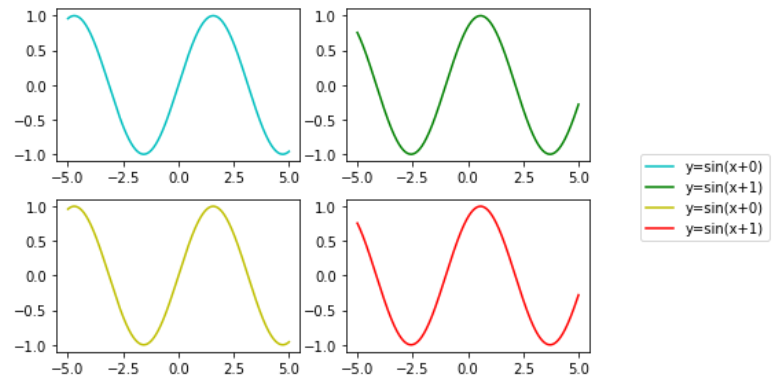My Personal Notes arrow_drop_up
Related Articles